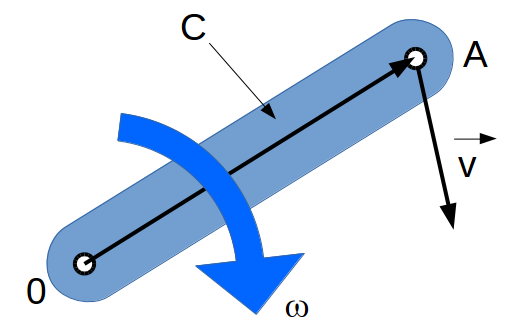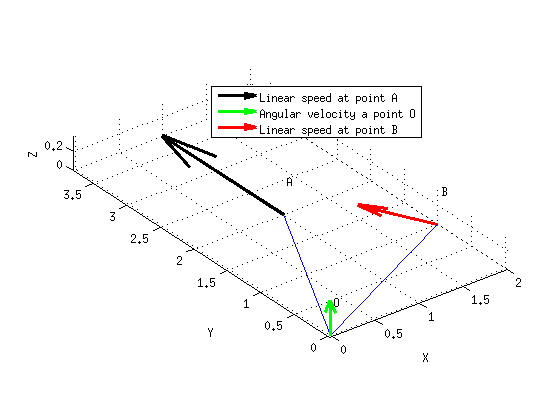Do me a favor, take a few seconds to have a look at my last project.

Thank you, Lulu

Angular and linear velocity, cross product

This page explains how to use the cross product to convert linear velocity to angular velocity (or the reverse).

From linear to angular

Let's consider the body C, rotating around the point O. The linear speed of point A is given by $$\vec{V}$$.The angular velocity around O is given by :

$$\vec{\omega} = \frac {\vec{OA} \times \vec{V}} { \| \vec{OA} \| ^2 }$$

From angular to linear

Assuming the angular velocity is known, the linear speed at point A is given by:

$$\vec{V} = \vec{\omega} \times \vec{OA}$$

ExampleThe following Matlab code shows how to convert linear velocity to angular velocity and vice-versa:

close all;
clear all;
clc;

%% Parameters

% Coordinates of point O
O=[0;0;0];
% Coordinates of point A
A=[1;2;0];
% Coordinates of point B
B=[2;1;0];

% Speed applied at point A
VA=[0;2;0];

%% Compute angular velocity

% Compute vector OA
vOA=A-O;
% Compute angular speed around point A
wO=cross (vOA,VA)/(norm(vOA)*norm(vOA));

%% Compute linear speed at point B

vOB=B-O;
VB=cross(wO,vOB);

%% Draw system

plot3(O(1),O(2),O(3));
hold on;
line ([O(1) A(1)],[O(2) A(2)],[O(3) A(3)]);
line ([O(1) B(1)],[O(2) B(2)],[O(3) B(3)]);
text(O(1)+0.2,O(2)+0.2,O(3)+0.2,'O','FontSize',100);
text(A(1)+0.2,A(2)+0.2,A(3)+0.2,'A','FontSize',100);
text(B(1)+0.2,B(2)+0.2,B(3)+0.2,'B','FontSize',100);

% Draw speed at point A

% Draw speed at point O

% Draw speed at point B